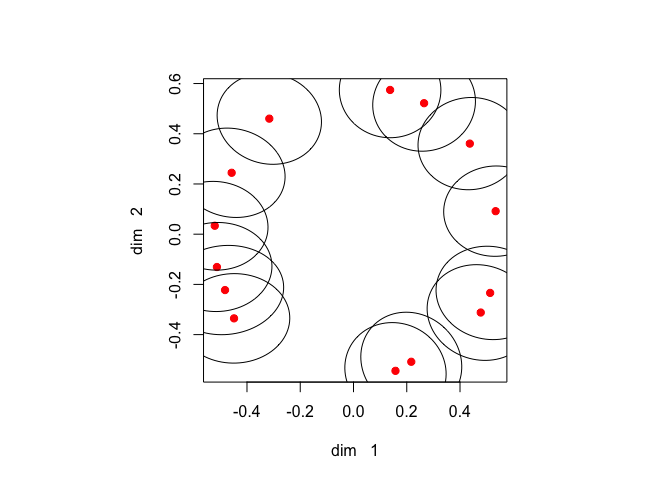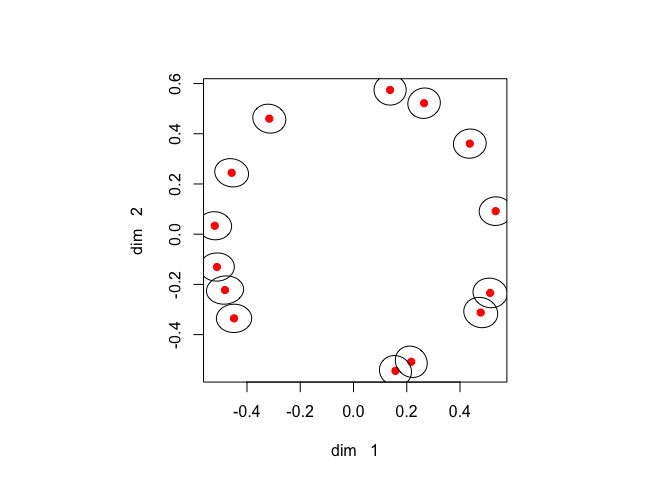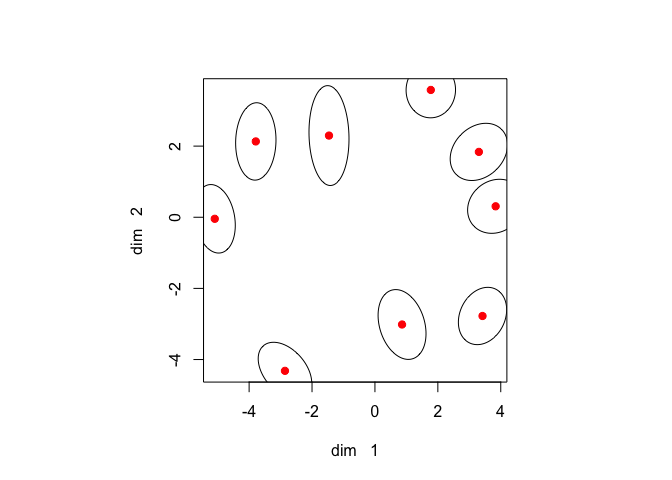Note: This is a working paper which will be expanded/updated frequently. All suggestions for improvement are welcome. The directory deleeuwpdx.net/pubfolders/confidence has a pdf version, the complete Rmd file with all code chunks, the R and C code, and the bib file.

# 1 Introduction

We briefly review the (metric, Euclidean, least squares) multidimensional scaling or MDS problem. We want to minimize the stress loss function $\sigma(x):=\frac12\mathop{\sum\sum}_{1\leq i < j\leq n}w_{ij}(\delta_{ij}-\sqrt{x'A_{ij}x})^2$ over $$x$$. Here $$x=\mathbf{vec}(X)$$, with $$X$$ the usual MDS configuration of $$n$$ points in $$p$$ dimensions. The distances between points $$i$$ and $$j$$ in the configurations are $$d_{ij}(x):=\sqrt{x'A_{ij}x}$$.

The $$np\times np$$ matrices $$A_{ij}$$ are defined using unit vectors $$e_i$$ and $$e_j$$, all zero except for one element that is equal to one. If you like, the $$e_i$$ are the columns of the identity matrix. Define $E_{ij}:=(e_i-e_j)(e_i-e_j)',$ and use $$p$$ copies of $$E_{ij}$$ to make the direct sum $A_{ij}:=\underbrace{E_{ij}\oplus\cdots\oplus E_{ij}}_{p\text{ times}}.$ With this notation $\mathcal{D}\sigma(x)=Vx-B(x)x,$ where $B(x):=\mathop{\sum\sum}_{1\leq i < j\leq n}w_{ij}\frac{\delta_{ij}}{d_{ij}(x)}A_{ij},$ and $V:=\mathop{\sum\sum}_{1\leq i < j\leq n}w_{ij}A_{ij}$ Also $\mathcal{D}^2\sigma(x)=V-H(x),$ with $H(x):=\mathop{\sum\sum}_{1\leq i < j\leq n}w_{ij}\frac{\delta_{ij}}{d_{ij}(x)}\left\{A_{ij}-\frac{A_{ij}xx'A_{ij}}{x'A_{ij}x}\right\}.$

# 2 Confidence Regions

Elliptical confidence regions for bivariate statistics are usually derived by using the dispersion matrix of the asymptotic normal distribution of a suitably normed version of the statistic. Equivalently, in the case of a log likelihood loss function, we can also use the observed or expected values of the Hessian of the loss.

There have been various attempts to put multidimensional scaling on a firm, or at least less shaky, statistical footing. Most notably, there are the papers by Ramsay (1977) and Ramsay (1982), which assume a parametric residual model of the form $$\underline{\delta}_{ij}\sim d_{ij}(X)\underline{\xi}_{ij}$$, i.e. $$\log\underline{\delta}_{ij}\sim \log d_{ij}(X)+\log\underline{\xi}_{ij}$$, and then apply likelihood theory. This may be applicable for some forms of dissimilarity measurements, but clearly not if the data are counts, rank orders, or transformed frequencies. Or if the notions of independence and repeated trials do not make sense.

A somewhat reasonable non-statistical technique to measure stability was proposed by De Leeuw and Meulman (1986). They developed a specialized leave-one-out method that can be used both in metric and non-metric multidimensional scaling. This MDS-jackknife, which visualizes stability by star-graphs with $$n$$ leaves around the $$n$$ points of the MDS solution, is incorporated in recent versions of the smacof package (De Leeuw and Mair (2009)).

In this paper we also take the point of view that we want to visualize stability without assuming a particular model. If $$y$$ is a solution of the MDS problem, i.e. a local minimum with $$\mathcal{D}\sigma(y)=0$$, then choose an $$\epsilon>0$$ and look at the region $\mathcal{S}_\epsilon(y):=\{x\mid\frac{\sigma(x)-\sigma(y)}{\sigma(y)}<\epsilon\},$ or the region $\mathcal{T}_\epsilon(y):=\{x\mid\sigma(x)-\sigma(y)<\epsilon\}.$ The size of the region will indicate how much $$y$$ can change without much change in $$\sigma()$$, and the shape of the region will indicate in which directions we can expect the largest change.

In general we will tend to use the relative region $$\mathcal{S}_\epsilon(y)$$ if $$\sigma(y)$$ is moderate or large, and the absolute region $$\mathcal{T}_\epsilon(y)$$ is $$\sigma(y)$$ is really small. As a strategy it makes sense to first do the MDS analysis, and then use the obtained local minimum value $$\sigma(y)$$ to choose $$\epsilon$$.

# 3 Analysis

At a local minimum $$y$$ $\sigma(x)\approx\sigma(y)+\frac12(x-y)'\mathcal{D}^2\sigma(y)(x-y),$ and thus $\begin{equation}\label{E:seps} \mathcal{S}_\epsilon(y)\approx\{x\mid(x-y)'\mathcal{D}^2\sigma(y)(x-y)\leq 2\epsilon\sigma(y)\}, \end{equation}$ and $\begin{equation}\label{E:teps} \mathcal{T}_\epsilon(y)\approx\{x\mid(x-y)'\mathcal{D}^2\sigma(y)(x-y)\leq 2\epsilon\} \end{equation}$ If we only vary the location of point $$i$$ in the configuration, then $$x-y$$ has only two non-zero elements, and we only use a $$2\times 2$$ principal submatrix of $$\mathcal{D}^2\sigma(y)$$ to plot the ellipse in $$\eqref{E:seps}$$ and $$\eqref{E:teps}$$. By varying $$i$$, using different principal submatrices of $$\mathcal{D}^2\sigma(y)$$, we get $$n$$ different ellipses around each point of the configuration.

# 4 Implementation

We use the smacof implementation, and the computation of the Hessian at the solution, from De Leeuw (2017). The smacofEllipseRC() function in the appendix does a smacof analysis, makes the plot of the configuration in two selected dimensions, and draws pseudo-confidence ellipses of a given radius around each point. As usual, ellipses are plotted by creating a large number of points equally spaced on the unit circle, then transforming them linearly so that they are on the appropriate ellipse, and then connecting them.

# 5 Examples

## 5.1 Ekman

Unavoidably, we first illustrate our ellipses by using the color data from Ekman (1954). The smacof analysis in two dimensions takes 70 iterations to come up with stress 0.5278528185. With an absolute $$\epsilon$$ of .1 we draw ellipses that show how points can be moved around while keep stress below 0.6278528185.Figure 1: Ekman with Absolute Eps .1

If we change $$\epsilon$$ to .01 then the configuration stays the same, the shape of the ellipses stays the same, they just become uniformly smaller.Figure 2: Ekman with Absolute Eps .01

## 5.2 De Gruijter

The second example are dissimilarity ratings between Dutch political parties in 1967, averaged over 50 students, and taken from De Gruijter (1967).

##       KVP PvdA  VVD  ARP  CHU  CPN  PSP   BP
## PvdA 5.63
## VVD  5.27 6.72
## ARP  4.60 5.64 5.46
## CHU  4.80 6.22 4.97 3.20
## CPN  7.54 5.12 8.13 7.84 7.80
## PSP  6.73 4.59 7.55 6.73 7.08 4.08
## BP   7.18 7.22 6.90 7.28 6.96 6.34 6.88
## D66  6.17 5.47 4.67 6.13 6.04 7.42 6.36 7.36

A smacof analysis in two dimensions takes 1283 iterations to come up with stress 32.2208145298. We use an absolute region, with $$\epsilon=1$$, i.e. we show how points can be moved around while keeping stress less than 33.2208145298.Figure 3: De Gruijter with Absolute Eps 1

# 6 Code

smacofEllipseRC <-
function (s, t, w, delta, p, eps, relative = FALSE) {
m <- length (w)
n <- round ((1 + sqrt (1 + 8 * m)) / 2)
r <- n * p
hsma <- smacofRCU(w, delta, p)
eps <- ifelse (relative, 2 * hsma$s * eps, 2 * eps) hvec <- smacofHessianRC(hsma$x, hsma$b, smacofVmatRC (w), hsma$d, w, delta)
xmat <- matrix (hsma$x, n, p) plot ( xmat, col = "RED", pch = 21, bg = "RED", xlab = paste("dim ", formatC( s, digits = 1, format = "d" )), ylab = paste("dim ", formatC( t, digits = 1, format = "d" )) ) hmat <- matrix (trimatRC(hvec), r, r) z <- cbind (sin (seq(-pi, pi, length = 100)), cos (seq(-pi, pi, length = 100))) * sqrt(eps) for (i in 1:n) { ii <- c((s - 1) * n + i, (t - 1) * n + i) y <- xmat[i, c(s, t)] amat <- c(hmat[ii, ii], hmat[ii, ii], hmat[ii, ii]) hjac <- jacobi (amat) zi <- z %*% diag (1 / sqrt (hjac$values))
zi <- tcrossprod(zi, hjac$vectors) zi <- zi + matrix (y, 100, 2, byrow = TRUE) lines (list(x = zi[, 1], y = zi[, 2])) } return ( list ( x = xmat, dist = hsma$d,
bmat = hsma$b, hessian = hmat, stress = hsma$s,
itel = hsma\$itel
)
)
}